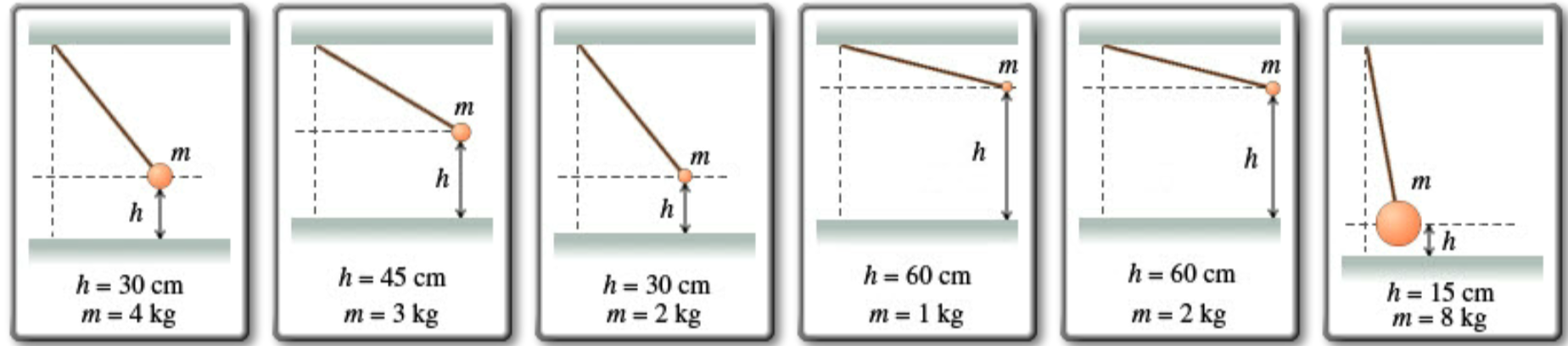# Problem: Six pendulums of various masses m are released from various heights h above a tabletop, as shown in the figures below. All the pendulums have the same length and are mounted such that at the vertical position their lowest points are the height of the tabletop and just do not strike the tabletop when released. Assume that the size of each bob is negligible. a) Rank each pendulum on the basis of the maximum kinetic energy it attains after release. b) Rank each pendulum on the basis of its maximum speed. Rank from largest to smallest. To rank items as equivalent, overlap them.

###### FREE Expert Solution

a) Law of conservation of energy:

$\overline{){\mathbf{P}}{\mathbf{.}}{{\mathbf{E}}}_{{\mathbf{0}}}{\mathbf{+}}{\mathbf{K}}{\mathbf{.}}{{\mathbf{E}}}_{{\mathbf{0}}}{\mathbf{=}}{\mathbf{P}}{\mathbf{.}}{{\mathbf{E}}}_{{\mathbf{f}}}{\mathbf{+}}{\mathbf{K}}{\mathbf{.}}{{\mathbf{E}}}_{{\mathbf{f}}}}$

Kinetic energy

$\overline{){\mathbf{K}}{\mathbf{.}}{\mathbf{E}}{\mathbf{=}}\frac{\mathbf{1}}{\mathbf{2}}{\mathbf{m}}{{\mathbf{v}}}^{{\mathbf{2}}}}$

$\begin{array}{rcl}\mathbf{m}\mathbf{g}\mathbf{h}& \mathbf{=}& \frac{\mathbf{1}}{\mathbf{2}}\mathbf{m}{{\mathbf{v}}_{\mathbf{f}}}^{\mathbf{2}}\end{array}$

For pendulum 1:

K.E1 = mgh = (4)(9.81)(30 × 10-2) = 11.8 J

For pendulum 2:

K.E2 = mgh = (3)(9.81)(45 × 10-2) = 13.2 J

For pendulum 3:

K.E3 = mgh = (2)(9.81)(30 × 10-2) = 5.9 J

For pendulum 4:

92% (251 ratings)###### Problem Details

Six pendulums of various masses m are released from various heights h above a tabletop, as shown in the figures below. All the pendulums have the same length and are mounted such that at the vertical position their lowest points are the height of the tabletop and just do not strike the tabletop when released. Assume that the size of each bob is negligible.a) Rank each pendulum on the basis of the maximum kinetic energy it attains after release.

b) Rank each pendulum on the basis of its maximum speed. Rank from largest to smallest. To rank items as equivalent, overlap them.